学哥量化交易学习 持续学习，提高认知，磨炼技艺，从零基础学编程并跨过量化程序交易的大门。 返回首页

0007 编程入门python语言之四则运算器

```num1=input("num1:")
num2=input("num2:")
num3=num1+num2
print num1,"+",num2,"=",num3

num1=input("num1:")
num2=input("num2:")
num3=num1-num2
print num1,"-",num2,"=",num3

num1=input("num1:")
num2=input("num2:")
num3=num1*num2
print num1,"*",num2,"=",num3

num1=input("num1:")
num2=input("num2:")
num3=num1/num2
print num1,"/",num2,"=",num3

0和1的世界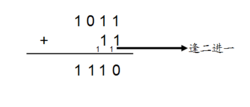not   逻辑非   不是
and 逻辑与   并且
or     逻辑或   或者

not 相反的判断，针对1个逻辑值计算

and 两者都为True则结果为True否则为False，针对2个逻辑值计算

or 两者任一为True则结果为True，两者都是False才为False，针对2个逻辑值计算

，结果为True，最后计算False and True，最后结果为False

if判断语句

if 逻辑运算结果1=True :
执行A
elif 逻辑运算结果2=True :
执行B
else :
执行C

python最重要的规则：缩进的空格数整个程序必须一致。

if判断语句举例：

if score>=60 :
print "及格"

if score>=60 :
print "及格"
else :
print "不及格"

if score>=80 :
print "良好"
elif score>=60 :
print "及格"
else :
print "不及格"

if score>=90 :
print "优秀"
elif score>=80 :
print "良好"
elif score>=60 :
print "及格"
else :
print "不及格"

if score==100 :
print "满分"
elif score>=90 :
print "优秀"
elif score>=80 :
print "良好"
elif score>=60 :
print "及格"
else :
print "不及格"

if判断输入的操作符号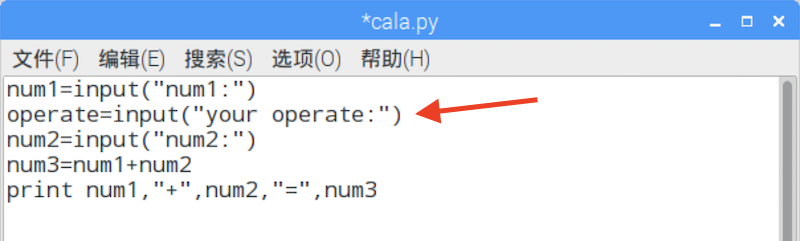if operate=="+" :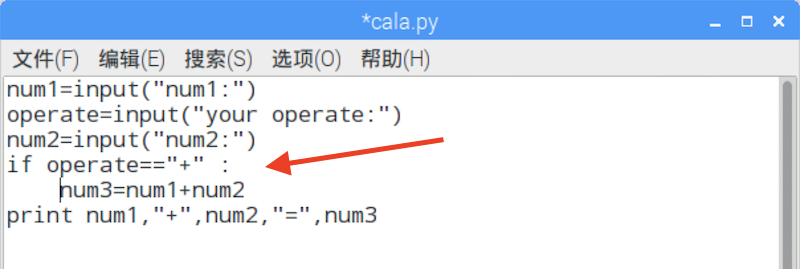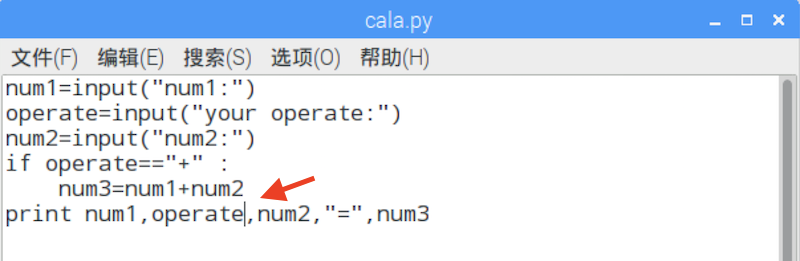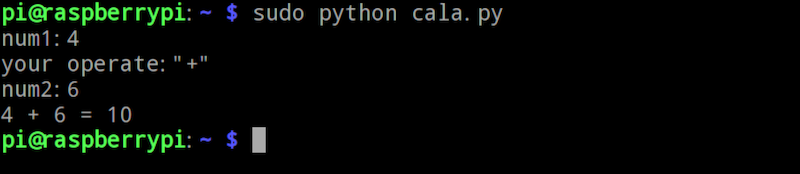elif operate=="-" :
num3=num1-num2
elif operate=="*" :
num3=num1*num2
elif operate=="/" :
num3=num1/num2
else :
num3="error operate"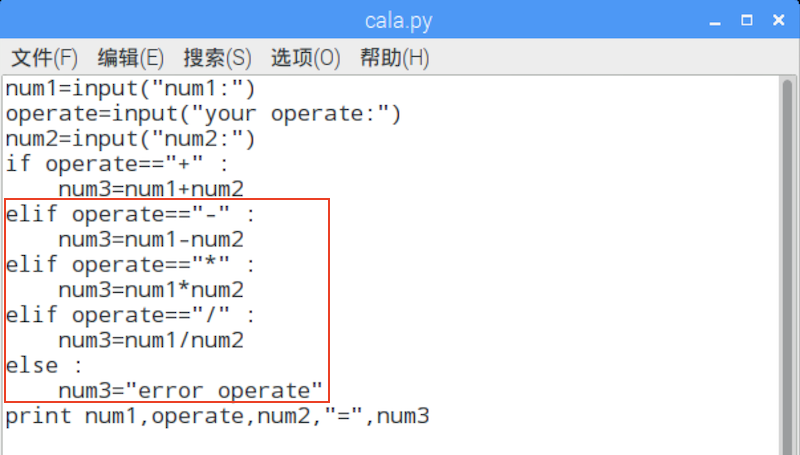1.确保程序本身正确执行，不会崩溃或死循环，或者无法执行
2.确保程序是按照设计和预期进行执行，得到的结果是正确和符合预期的
3.确保程序在不同环境或者不同条件情况下，仍然能够正常工作，当然这些条件都是在设计之中。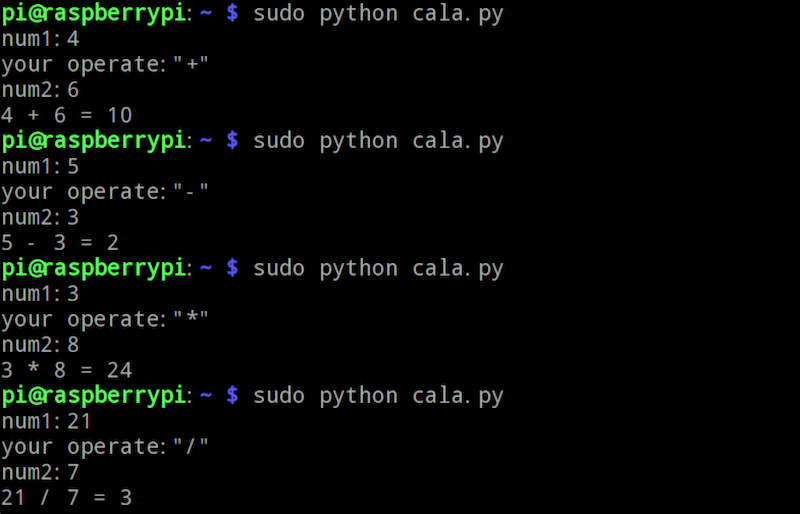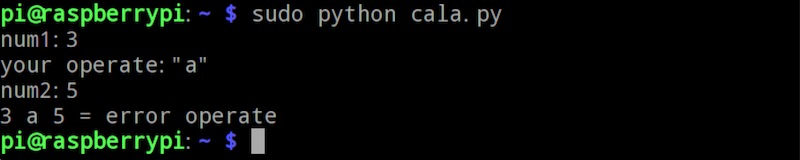```学哥量化交易学习团队 致力于：持续学习，提高认知，磨炼技艺，持续输出高质量的教程和课程，帮助更多的人从零基础学编程并跨过量化程序交易的大门。 零基础学编程Python入门课程 课程包含以下内容： 1.图文教程《零基础学编程入门-从Python到Web网站.pdf》，学哥原创版权，共计1024页高清图文教程。 2.视频课程《零基础学编程Python入门》，学哥原创版权，共计24节课45个高清视频。 3.视频教程《玩转魔方》，学哥原创版权，可以送给孩子，共计16个高清视频。 4.图文教程《如何寻找编程相关的电子书下载资源.pdf》。 5.加入学哥编程学习辅导QQ群在线交流。 客服 10:00-17:00QQ: 44556287 微信公众号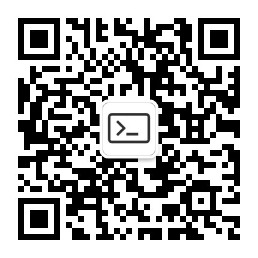学哥量化交易学习

 微信公众号：学哥量化交易学习     QQ群1：603559164    客服QQ号：44556287 工信部备案/许可证编号：沪ICP备15050545号-4